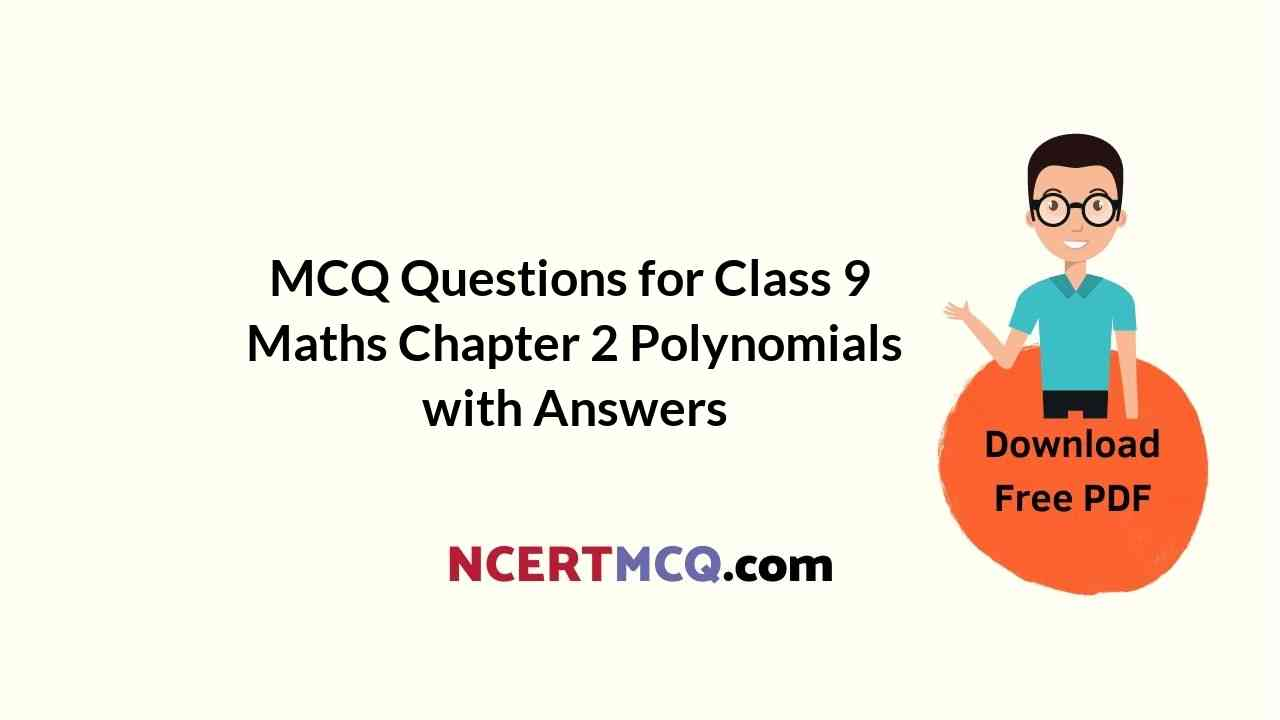Check the below Online Education NCERT MCQ Questions for Class 9 Maths Chapter 2 Polynomials with Answers Pdf free download. MCQ Questions for Class 9 Maths with Answers were prepared based on the latest exam pattern. We have provided Polynomials Class 10 Maths MCQs Questions with Answers to help students understand the concept very well. https://ncertmcq.com/mcq-questions-for-class-9-maths-with-answers/

Students can also refer to NCERT Solutions for Class 9 Maths Chapter 2 Polynomials for better exam preparation and score more marks.

## Online Education for Polynomials Class 9 MCQs Questions with Answers

Polynomials Class 9 MCQ Question 1.
Find the product of (x – $$\frac{1}{x}$$) (x + $$\frac{1}{x}$$) (x² + $$\frac{1}{x^2}$$)
(a) x4 + $$\frac{1}{x^4}$$
(b) x³ + $$\frac{1}{x^3}$$ – 2
(c) x4 – $$\frac{1}{x^4}$$
(d) x² + $$\frac{1}{x^2}$$ + 2

Answer: (c) x4 – $$\frac{1}{x^4}$$

Class 9 Maths Chapter 2 MCQ Question 2.
If $$\frac{x}{y}$$ + $$\frac{y}{x}$$ = -1 (x, y ≠ 0), then find the value of x³ – y³
(a) 1
(b) -1
(c) $$\frac{1}{2}$$
(d) 0

Polynomial Class 9 MCQ Question 3.
Find the value of 525² – 475².
(a) 100
(b) 10000
(c) 50000
(d) 100000

Class 9 Maths Chapter 2 MCQ With Answers Question 4.
One of the factors of (1 + 3y)² + (9y² – 1) is
(a) 1 – 3y
(b) 3 – y
(c) 3y + 1
(d) y – 3

Is this a polynomial calculator can help you find a polynomial curve that best fits your data set.

MCQ Of Polynomials Class 9 Question 5.
One of the factors of (x³ – 1) – (x – 1) is
(a) x² + 1
(b) x² – 1
(c) x – 1
(d) x + 4

Class 9 Polynomials MCQ Question 6.
Find the degree of polynomial √2 .
(a) 2
(b) 0
(c) 1
(d) $$\frac{1}{2}$$

Polynomial MCQ Class 9 Question 7.
Find the value of k if x² + kx + 6 = (x + 2) (x + 3) for all k.
(a) 1
(b) -1
(c) 5
(d) 3

Polynomials MCQ Class 9 Question 8.
If x – 2 is a factor of 5x² – kx – 18, then find the value of k.
(a) -1
(b) 1
(c) 0
(d) 5

This Calculator computes the Degree and Leading Coefficient Calculator term of a given Polynomial.

Class 9 Maths Ch 2 MCQ Question 9.
Find the coefficient of x² in (3x² – 5) (4 + 4x²).
(a) 12
(b) 5
(c) -8
(d) 8

Chapter 2 Maths Class 9 MCQ Question 10.
Find the value of p for which x + p is a factor of x² + px + 3 – p.
(a) 1
(b) -1
(c) 3
(d) -3

Ch 2 Maths Class 9 MCQ Question 11.
Zero of the polynomial p(x) = cx + d is
(a) -d
(b) -c
(c) $$\frac{d}{c}$$
(d) –$$\frac{d}{c}$$

Answer: (d) –$$\frac{d}{c}$$

MCQ Questions For Class 9 Maths Chapter 2 Question 12.
Find the remainder on dividing 5y³ – 2y² – 7y + 1 by y.
(a) -1
(b) 1
(c) 0
(d) 2

MCQ Questions For Class 9 Maths Ncert Chapter 2 Question 13.
One of the factors of (16y² – 1) + (1 – 4y)²
(a) (4 + a)
(b) (4 – y)
(c) (4y + 1)
(d) 8y

Class 9 Chapter 2 MCQ Question 14.
If a + b + c = 0, then the value of a³ + b³ + c³
(a) abc
(b) -3abc
(c) 0
(d) 3abc

Class 9 Math Chapter 2 MCQ Question 15.
If x$$\frac{1}{3}$$ + y$$\frac{1}{3}$$ + z$$\frac{1}{3}$$ = 0, then which one of the expression is correct.
(a) x³ + y³ + z³ = 0
(b) x + y + z = 3x$$\frac{1}{3}$$y$$\frac{1}{3}$$z$$\frac{1}{3}$$
(c) x + y + z = 3xyz
(d) x³ + y³ + z³ = 3xyz

Answer: (b) x + y + z = 3x$$\frac{1}{3}$$y$$\frac{1}{3}$$z$$\frac{1}{3}$$

Question 16.
If the area of the rectangle is 4x² + 4x – 3, then find its possible dimensions.
(a) 2x – 3, 2x + 1
(b) 2x – 1, 2x + 3
(c) 3x + 1, 2x – 3
(d) 3x – 1, 2x + 3

Answer: (b) 2x – 1, 2x + 3

Question 17.
If x + $$\frac{1}{x}$$ = 4, then the value of x² + $$\frac{1}{x^2}$$
(a) 8
(b) 14
(c) 16
(d) 20

Question 18.
For what value of b, is the polynomial x³ – 3x² + bx – 6 divisible by x – 3?
(a) 1
(b) 2
(c) 3
(d) -3

Question 19.
When p(x) is divided by ax – b, then the remainder is
(a) p(a + b)
(b) P($$\frac{-b}{a}$$)
(c) p($$\frac{a}{b}$$)
(d) p($$\frac{b}{a}$$)

Answer: (d) p($$\frac{b}{a}$$)

Question 20.
What is remainder when x³ – 2x² + x + 1 is divided by x – 1?
(a) 0
(b) -1
(c) 1
(d) 2